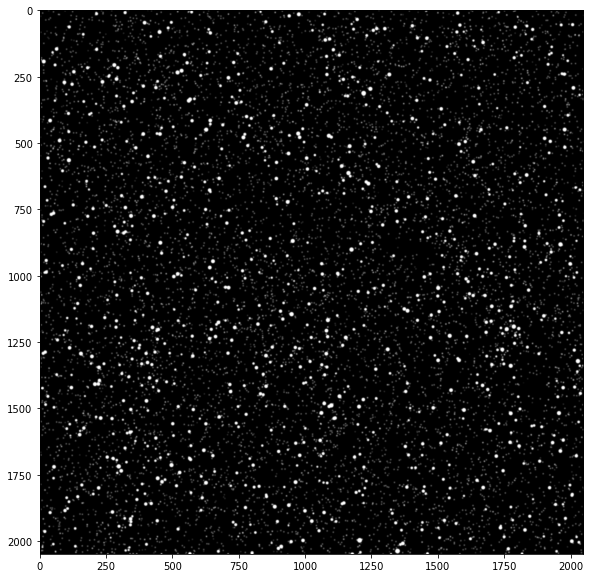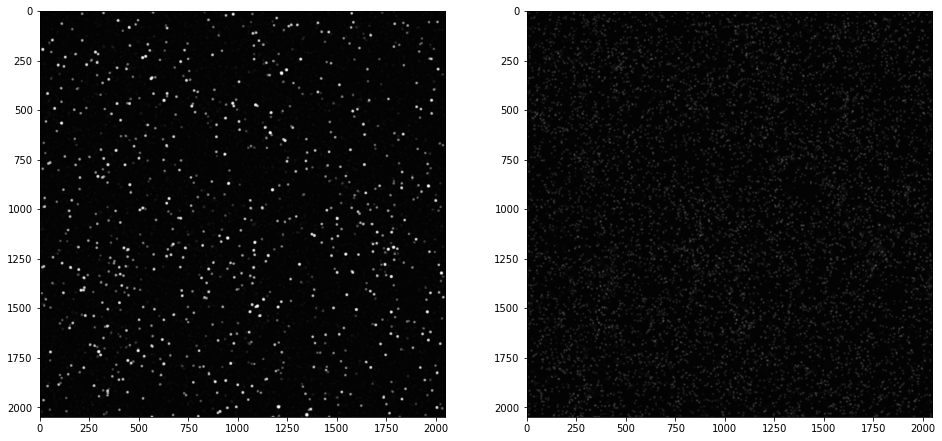# Phase separation tutorial¶

In the first section, we load an artificial two-phase PIV image and then use different phase separation methods to discriminate the two phases and save them as separate images. This section aims to introduce different methods that are available.

In the second section, we demonstrate phase separation on real images followed by actual PIV process as a more realistic example.

# Section 1 - Introducing different methods¶

## Loading image¶

In :
from openpiv import tools, phase_separation
import matplotlib.pyplot as plt
plt.gray()

<Figure size 432x288 with 0 Axes>
In :
two_phase_image = tools.imread('../two_phase_piv/artificial_A.tif')
plt.figure(figsize=(10,10))
plt.imshow(two_phase_image)
plt.show()## Opening method¶

In :
big_particles_image, small_particles_image = phase_separation.opening_method( two_phase_image, 11, thresh_factor=1.05 )
fig, ax = plt.subplots(1, 2, figsize=(16,10))
ax.imshow( big_particles_image, vmin=0, vmax=255 )
ax.imshow( small_particles_image, interpolation='bicubic', vmin=0, vmax=255 )
plt.show()## Median filter method¶

In :
big_particles_image, small_particles_image = phase_separation.median_filter_method(two_phase_image, 11)
fig, ax = plt.subplots(1, 2, figsize=(16,10))
ax.imshow( big_particles_image)
ax.imshow( small_particles_image, vmin=0, vmax=255 )
plt.show()